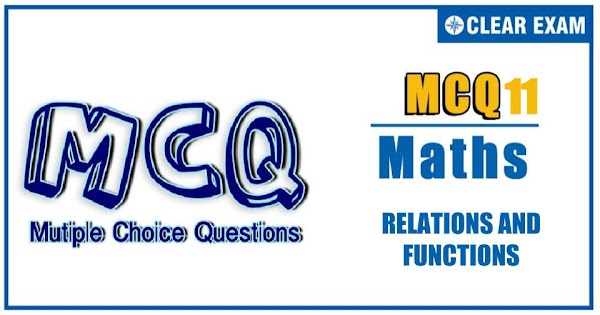## RELATIONS AND FUNCTIONS Quiz-11

In mathematics, a function can be defined as a rule that relates every element in one set, called the domain, to exactly one element in another set, called the range. For example, y = x + 3 and y = x2 – 1 are functions because every x-value produces a different y-value. A relation is any set of ordered-pair numbers..

Q1. The total number of solutions of [x]^2=x+2{x}, where [.] and {.} denote the greatest integer function and fractional part, respectively, is equal to
•  2
•  4
•  6
•  None of these
Solution
(b) [x]^2=x+2{x} ⇒[x]^2=x+3{x} ⇒{x}=([x]^2-[x])/3 ⇒x=-1/3,0,1,8/3

Q2.The domain of definition of the function y=1/log_10⁡〖(1-x)〗 +√(x+2)=
•  (-3, -2) excluding -2.5
•  [0,1] excluding 0.5 >
•   [-2,1) excluding 0
•  None of these
Solution
(c) y=1/log_10⁡(1-x) +√(x-2) y=f(x)+g(x) Then, the domain of given function is D_f∩D_g Now, for the domain of f(x)=1/log_10⁡〖(1-x)〗 , We know it is defined only when 1-x>0 and 1-x≠1

Q3.  Given the function f(x)=(a^x +〖 a〗^(-x))/2 (where a>2). Then f(x+y)+f(x-y)=
•   2f(x).f(y)
•  f(x).f(y)
•  (f(x))/(f(y))
•  None of these
Solution
(d) We have fog(x)=f(g(x))=sin⁡〖(log_e⁡|x|)〗 log_e⁡|x| has range R, for which sin⁡(log_e⁡|x| )ϵ[-1,1] ∴R_1={u:-1≤u≤1} Also gof(x)=g(f(x))=log_e⁡|sin⁡x | ∵0≤|sin⁡x |≤1

Q4. Let f(x)=([a]^2-5[a]+4) x^3-(6{a}^2-5{a}+1)x-(tan⁡x )×sgn x be an even function for all x∈R, then the sum of all possible values of 'a' is (where [⋅] and {⋅} denote greatest integer function and fractional part functions, respectively)
•  17/6
•  53/6
•  31/3
•  35/3
Solution
(d) f(x)=〖αx〗^3-βx-(tan⁡x )sgn x f(-x)=f(x) ⇒-〖αx〗^3+βx-tan⁡〖x sgn x=〗 〖αx〗^3-βx-(tan⁡x )(sgn x) ⇒2(-〖αx〗^2-β)x=0∀x∈R ⇒α=0 and β=0 ∴〖[a]〗^2-5[a]+4=0 and 6〖{a}〗^2-5{a}+1=0 ⇒(3{x}-1)(2{x}-1)=0 ∴a=1+1/3,1+1/2,4+1/3,4+1/2 Sum of values of a=35/3

Q5.The range of the function f defined by f(x)=[1/sin⁡{x} ] (where [.] and {.} respectively denote the greatest integer and the fractional part functions) is
•  I, the set of integers
•  N, the set of natural numbers
•  W, the set of whole numbers
•  {1, 2, 3, 4,…}
Solution
(d) ∵{x}∈[0,1) sin⁡x∈(0,sin⁡1 ) as f(x) is defined if sin⁡〖{x}≠0〗 ⇒1/sin⁡{x} ∈(1/sin⁡1 ,∞)⇒[1/sin⁡{x} ]∈{1,2,3,…}

Q6. The period of the function f(x)=[6x+7]+cos⁡〖πx-6x〗, where [.] denotes the greatest integer function, is
•  3
•
• 2
•  None of these
Solution
(c) f(x)=[6x+7]+cos⁡〖πx-6x〗 =[6x]+7+cos⁡〖πx-6x〗 =7+cos⁡〖πx-{6x〗} {6x} has the period 1/6 and cos⁡πx has the period 2, then the period of f(x)= LCM of 2 and 1/6 which is 2 Hence, the period is 2

Q7. If f(x+y)=f(x).f(y) for all real x,y and f(0)≠0, then the function g(x)=f(x)/(1+{f(x)}^2 ) is
•  Even function
•  Odd function
•  Odd if f(x)>0
•  Neither even nor odd
Solution
(a) Given f(x+y)=f(x)f(y) Put x=y=0, then f(0)=1 Put y=-x, then f(0)=f(x)f(-x)⇒f(-x)=1/f(x) Now, g(x)=f(x)/(1+{f(x)}^2 ) ⇒ g(-x) f(-x)/(1+{f(-x)}^2 )=(1/f(x) )/(1+1/{f(x)}^2 ) =f(x)/(1+{f(x)}^2 )=g(x)

Q8. A real-valued function f(x) satisfies the functional equation f(x-y)=f(x)f(y)-f(a-x)f(a+y), where a is a given constant and f(0)=1.f(2a-x) is equal to
•  f(x)
•  T-f(x)
•  f(-x)
•  f(a)+f(a-x)
Solution
(b) We have f(x-y)=f(x)f(y)-f(a-x)f(a+y) Putting x=a and y=a-x, we get f(a-(x-a)=f(a)f(x-a)-f(0)f(x) (1) Putting x=0, y=0, we get f(0)=f(0)(f(0))-f(a)f(a) ⇒f(0)=(f(0))^2-(f(a))^2 ⇒1=〖(1)〗^2-(f(a))^2 ⇒f(a)=0 ⇒ f(2a-x)=-f(x)

Q9. If f and g are one-one function, then
•  f+g is one-one
•   f g is one-one
•  fog is one-one
•  None of these
Solution
(c) (a)f(x)=sin⁡x and g(x)=cos⁡x,x∈[0,π⁄2] Here, both f(x) and g(x) are one-one functions But h(x)=f(x)+g(x)=sin⁡x+cos⁡x is many-one as h(0)=h(π⁄2)=1 (b) h(x)=f(x)g(x)=sin⁡x cos⁡x=sin⁡2x/2 is many-one, as h(0)=h(π⁄2)=0 (c)It is a fundamental property

•  f_4 (x)=f_1 (x) for all x
•  f_1 (x)=-f_3 (-x) for all x
•  f_2 (-x)=f_4 (x) for all x
• f_1 (x)+f_3 (x)=0 for all x
Solution
B#### Written by: AUTHORNAME

AUTHORDESCRIPTION## Want to know more

Please fill in the details below:

## Latest NEET Articles\$type=three\$c=3\$author=hide\$comment=hide\$rm=hide\$date=hide\$snippet=hide

Name

ltr
item
BEST NEET COACHING CENTER | BEST IIT JEE COACHING INSTITUTE | BEST NEET & IIT JEE COACHING: Relations and Functions-Quiz-11
Relations and Functions-Quiz-11
https://1.bp.blogspot.com/-4_L67swRdnM/YOSDMwlmXwI/AAAAAAAAJB4/dHVBedcsQGAaSbEavAnyt0WhHDApsF9gwCLcBGAsYHQ/s600/Quiz%2BImage%2B20.jpg
https://1.bp.blogspot.com/-4_L67swRdnM/YOSDMwlmXwI/AAAAAAAAJB4/dHVBedcsQGAaSbEavAnyt0WhHDApsF9gwCLcBGAsYHQ/s72-c/Quiz%2BImage%2B20.jpg
BEST NEET COACHING CENTER | BEST IIT JEE COACHING INSTITUTE | BEST NEET & IIT JEE COACHING
https://www.cleariitmedical.com/2021/07/relations-and-functions-quiz-11.html
https://www.cleariitmedical.com/
https://www.cleariitmedical.com/
https://www.cleariitmedical.com/2021/07/relations-and-functions-quiz-11.html
true
7783647550433378923
UTF-8

STAY CONNECTED